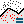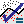Next Previous Up Contents
Next: Distance Measurement
Up: Defining Subsets Graphically
Previous: Draw Blob Subset

#### A.4.2.3.4 Draw Algebraic Subset

The Draw Algebraic Subset () action allows you to select points in a region of the plot by clicking on points of your choice to mark out a shape. Different shapes such as polygons and circles are available, depending on the plot type. When complete, subsets will be defined with an algebraic expression which you can see and edit. This can be particularly useful (and a better option than the blob) if you want to refer to the subset outside of the context of the current session, for instance in a STILTS command or a published paper.

This action is currently only available in the Plane and Sky plot windows.Defining a subset by algebraic drawing. This shows use of mode Below in the Plane plot.

When you use this action to define a plot region, it operates in one of a number of inclusion modes, depending on the plot type. In all cases, you click on one or more points to define the boundaries of the region. The available modes are described at the end of this section.

Operation is as follows:

1. To start marking out a shape, hit thebutton in the toolbar, and a popup window will first ask you which inclusion mode you want to use. Alternatively, you can use one of the mode-specific sub-menu items in the Subsets|Draw Algebraic Subset menu to choose a mode without the extra popup.
2. Once in drawing mode, the toolbar button will appear with a checkmark over it (), and a little square marker will appear near the mouse pointer as long as it's over a suitable part of the plot. You can then click on the plotting area to mark the points, and the area thus defined (according to the mode you have chosen) will be shaded in grey. Each point you have clicked on to define the area is marked with the little square marker. The algebraic form of the expression for the points entered so far will be displayed at the bottom of the screen. A right-click (or Ctrl-click) will remove the most recently-added point.
3. When you've finished adding points, click on thebutton again. This will pop up the Multi Algebraic Subset Window, which displays the algebraic function corresponding to the region you have outlined, and offering to create a new subset (or, if there are multiple datasets plotted, several new subsets) from it.

The generated expression tries to be as compact and comprehensible as possible. Precision of the indicated points is determined from the pixel resolution of the plot, so literal numbers are not more unwieldy than they have to be.

The available inclusion modes depend on the plot type, as follows:

Plane Plot
• Polygon: Select at least three points to define a closed polygon. The selected region is inside it. The expression generated uses the function `isInside` from the Shapes class.
• Box: Defines a rectangle aligned with the axes from two opposite corners. The expression is just of the form `x>x0 && x<x1 && y>y0 && y<y1`.
• Aligned Ellipse: Defines an ellipse aligned with the plot axes. The first point selected defines the ellipse center, and the next point is on the radius. If later points are selected, they reset the radius. The expression generated is of the form `square((x-x0)/a) + square((y-y0)/b)<1`, using the `square` function from the Maths class (`square(x)=x*x`).
• Rotated Ellipse: Defines an ellipse with arbitrary alignment. The first point selected defines the ellipse center, the second point is the end of the primary radius, and the third point defines the extent of the secondary radius. If later points are selected they reset the secondary radius. The expression generated is of the form `square(a(x-x0)+b(y-y0))+square(c(x-x0)+d(y-y0))<1`, using the `square` function from the Maths class (`square(x)=x*x`).
• Below, Above, Left, Right: The points define a jagged line representing a continuous function of x (or y) which has, in general, a discontinuous derivative. If just one point is selected, all the points on the corresponding side of that single point are included. Line segments at either end of the line are considered to continue to infinity - it's easy to understand how this works by trying it out (or see the figure above). If only one point is selected, the expression generated is of the form `y<y0`, and for two points it has the form `y<m*x+c`. If there are more points, the special function `polyLine` from class Shapes is used.
Sky Plot
• Circle: The first point selected defines the center of a small circle on the sphere (cone), and the next point is on its radius. If later points are selected, they reset the radius. The expression generated uses the `skyDistance` function from class Sky.
• Ellipse: Defines an ellipse with arbitrary alignment. The first point selected defines the ellipse center, the second point is the end of the primary radius, and the third point defines the extent of the secondary radius. If later points are selected they reset the secondary radius. The expression generated uses the `inSkyEllipse` function from class Sky.
• Polygon: Select at least three points to define a closed polygon. The edges of the polygon are the minor arcs of great circles (geodesics on the sphere). The expression generated uses the `inSkyPolygon` function from class Sky.

Next Previous Up Contents
Next: Distance Measurement
Up: Defining Subsets Graphically
Previous: Draw Blob Subset

TOPCAT - Tool for OPerations on Catalogues And Tables